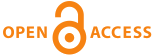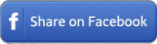Home|Journals|Articles by Year|Audio Abstracts RSS - TOCin any field in Title in Summary in Keywords in Authors in journals

 Technical NotePBS. 2012; 2(4): 191-193

#### Interpretation of correlation analysis results

Selim Kılıç.

##### Abstract

Correlation analysis is used to quantify the degree of linear association between two variables. Correlation coefficient is showed as “r” and it may have values between (-) 1 and (+)1. The symbols (-) or (+) in front of “r coefficient” show the direction of correlation. The direction of association does not affect the strength of association. A “ r coefficient” which is equal or greater than 0.70 is accepted as a good association. Correlation coeefficient only remarks the strength of association. Finding a good correlation coefficient between two variables does not explain a causal relationship between these two variables. Statistical significance is much affected from sample size in also correlation analysis. Square of corrrelation coefficient is described as coefficient of determination. Correlation coefficient is also much affected from outliers as standard deviation does. The proper test selection may vary depend on the type of variable and their distribution characteristics (ordinal, continuous, normally distributed or not). Pearson, Spearman and Kendall’s tau-B tests are the most common used tests. Partial correlation provides the explanation of the association between two variables when the third variable was controlled. When we interpret the correlation analysis results we firstly evaluate the strength of association and then indicate whether it is also statistically significant or not.

Key words: correlation, linear association, strength of association

 Share this ArticleOnline Article Submission
• ejmanager.com
• ojshosting.net